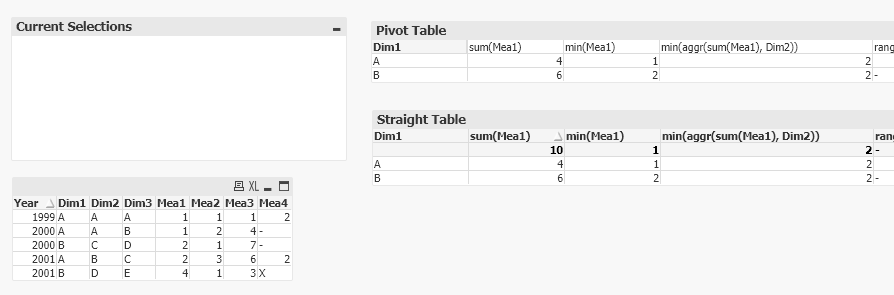# New to Qlik Sense

Discussion board where members can get started with Qlik Sense.

cancel
Showing results for
Did you mean:
HighlightedContributor III

## How to get functions like Stdev, Sum, Avg, etc. into rows in a table?

Hi,

Consider the following data:

X, Y
1, 10
2, 20
3, 30
4, 40
5, 50
];

The sum, avg, and stdev are as follows:  150, 30, & 15.8

I'd like a table that shows X, Y, sum, avg, stdev, X+Y, X+sum, and Y+stdev.  It should look like this:

I set X as a dimension
I set Y as a measure (I picked sum.  Does it matter?)
=sum(Y)
=avg(Y)
=stdev(Y)
=X+Y
=X+sum(Y)
=Y+stdev(Y)

What I want:

1   10   150   30   15.8   11   25.8
2   20   150   30   15.8   22   35.8
3   30   150   30   15.8   33   45.8
etc.

Instead, this is what I get:

1   10   10   10   -   11   11   -
2   20   20   20   -   22   22   -
3   30   30   30   -   33   33   -
etc.

Thanks!

1 Solution

Accepted Solutions
HighlightedSpecialist

## Re: How to get functions like Stdev, Sum, Avg, etc. into rows in a table?

Hi,

Where you have an aggregation across all of the Y values you need to use TOTAL, so your =sum(Y) should be =sum(TOTAL Y) etc.

Cheers,

Chris.

3 Replies
HighlightedSpecialist

## Re: How to get functions like Stdev, Sum, Avg, etc. into rows in a table?

Hi,

Where you have an aggregation across all of the Y values you need to use TOTAL, so your =sum(Y) should be =sum(TOTAL Y) etc.

Cheers,

Chris.

HighlightedContributor III

## Re: How to get functions like Stdev, Sum, Avg, etc. into rows in a table?

Thanks!

Would you have any insight on how aggr might be appropriate?  That's another commonly used function that I'm trying to understand.

Specifically I'm looking at this function that creates a reference line in a chart:

=avg(Y) + Stdev(total aggr(avg(Y),X))

EDIT:

This gives me exactly the same table as =Y + stdev(total Y) except that the above equation gives me some sort of average.

I'm still tinkering with it........

EDIT #2:

Okay, putting avg in fromt of Y give me the exact same equations.  So I'm not sure what aggr is accomplishing.

=Avg(Y) + stdev(total Y)
=Avg(Y) + stdev(total aggr(avg(Y),X))

HighlightedSpecialist

## Re: How to get functions like Stdev, Sum, Avg, etc. into rows in a table?

Hi,

Generally you will only see effects with AGGR that will help you understand how to use it when you have more than 1 dimension, otherwise you are only aggregating over the dimension you have.

You may or may not find the below helpful. Personally so I don't get drawn in to the data I quite like to set up toy examples with very simple data that I can point to/print out/scribble on. This one I did when I was trying to 'revise' AGGR for the QlikView BA exam I did a while ago (I'd used it in work, but quite a while before and for me it is easy to get rusty on AGGR).

So in the below the function min(aggr(sum(Mea1), Dim2)) means the minimum value of sum(Mea1) aggregated over Dim2, but for the Dim1 of each row (as there is no TOTAL in there).

So for Dim1=A, you can have Dim2=A or B, so you get 2 values;
Dim1=A & Dim2=A top 2 rows (table bottom left) - so sum is 2
Dim1=A & Dim2=B fourth row - so sum is 2 (again)
Hence min=2

For Dim1=B, you can have Dim2=C or D, so again 2 values;
Dim1=B & Dim2=C third row - so sum is 2
Dim1=B & Dim2=D fifth row - so sum is 4
Hence min=2I passed the exam, so I assume at least it was right in my head at the time 😀.

Cheers,
Chris